# Classifying Angles as Acute, Obtuse, Right or Reflex

Classifying Angles as Acute, Obtuse, Right or Reflex• Angles are classified by how many degrees they contain.
• An acute angle is less than 90°.
• A right angle is exactly 90°.
• An obtuse angle is larger than 90° but less than 180°.
• A straight line is exactly 180°.
• A reflex angle is larger than 180° but less than 360°
• A full turn is exactly 360°

Measure the size of an angle in degrees and compare this value to the table above to decide which category it is in..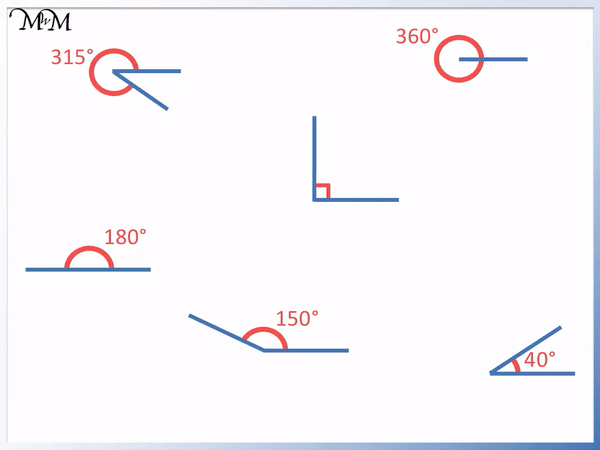• The first angle is 315° which is between 180° and 360°. It is a reflex angle.
• The second angle is exactly 90°. It is a right angle.
• The third angle is exactly 360°. It is a full turn.
• The fourth angle is exactly 180°. It is a straight line.
• The fifth angle is 150°, which is between 90° and 180°. It is an obtuse angle.
• The final angle is 40°, which is less than 90°. It is an acute angle.Supporting Lessons# Classifying Different Types of Angles

## What are the Different Types of Angle?

There are 7 main different types of angle:

• Zero Angles
• Acute Angles
• Right Angles
• Obtuse Angles
• Straight Lines
• Obtuse Angles
• Full Turns

The different types of angle are classified by their size in the table below.

Type of Angle Size in Degrees
Zero Angle Exactly 0°
Acute Angle Less than 90°
Right Angle Exactly 90°
Obtuse Angle Larger than 90° and less than 180°
Straight Angle Exactly 180°
Reflex Angle Larger than 180° and less than 360°
Full Turn Exactly 360°

## How to Classify an Angle

To classify an angle, first measure its size in degrees. Then compare this angle to the following values:

• If the angle is less than 90°, it is an acute angle.
• If the angle is exactly 90°, it is a right angle.
• If the angle is between 90° and 180°, it is an obtuse angle.
• If the angle is exactly 180°, it is a straight line.
• If the angle is exactly 360°, then it is a full turn.

Although you can turn through an angle that is larger than 360°, there is no new name for an angle that is larger than 360°.

For example, turning 360° completes ones full turn and completing 720° is two full turns because 2 lots of 360° is 720°.

Here is a summary table of the different angle types.Here are some examples of identifying angle types.The angle in the top left is 315°. This angle is larger than 180° but it is less than 360°. This means that it opens wider than a straight line but it is not quite a full turn. The angle is a reflex angle.

The angle in the top right shows a turn that has gone all the way around back to where it started. It shows a full turn. A full turn is 360°.

The angle in the middle is a right angle. This is because it is exactly 90°. It is also marked with a square in the corner of the angle which tells us that it is a right angle.The angle at the bottom left is a straight line. Straight lines are exactly 180°.

The angle in the bottom middle is 150°. 150° is larger than 90° but it is less than 180°. It is larger than a right angle but it is not as large as a straight line angle. This is an obtuse angle.

The angle in the bottom right is 40°. 40° is less than 90° and so, this angle is an acute angle.

Here is another example of classifying an angle.

This angle is 60°.This angle is less than 90° and so, this angle is classified as an acute angle.

Apart from a zero angle, there is no smaller angle than an acute angle. Since zero angles look like a straight line, they are difficult to work with and are not commonly found in typical angle classification questions.

Here is another example of identifying an angle type.

Here we have a 280° angle.280° is larger than a straight line angle of 180° and it is less than a full turn of 360°. It is a reflex angle.

It is important to make sure that you measure the correct angle when measuring reflex angles. We are measuring the marked arrow shown in red on the diagram. We have rotated all the way around past a straight line to get to this point.

## Acute Angles

Acute angles refer to any angle that is less than 90°. This means that an acute angle is smaller than a right angle.

Here are some examples of acute angles.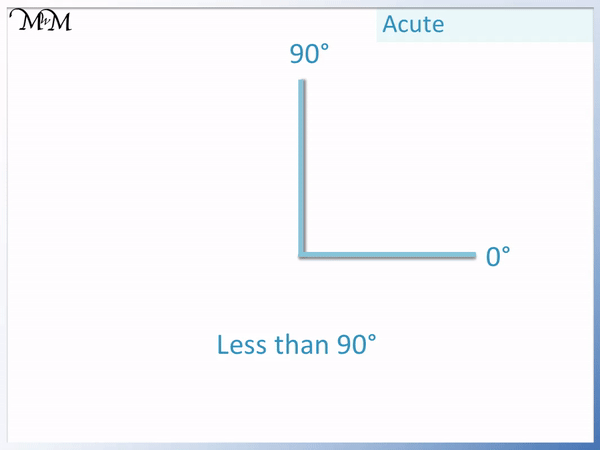When teaching acute angles, we can remember that these are the smallest type of angle classification and so, they are ‘a cute’ angle. Linking the idea of being small and being cute can make this name easy to remember.

Acute angles along with right angles are probably the easiest type of angles to learn first because they are most commonly seen in day-to-day life.

## Right Angles

Right angles measure exactly 90°. They are a quarter of a full turn and are shown with a small square in the corner of the angle.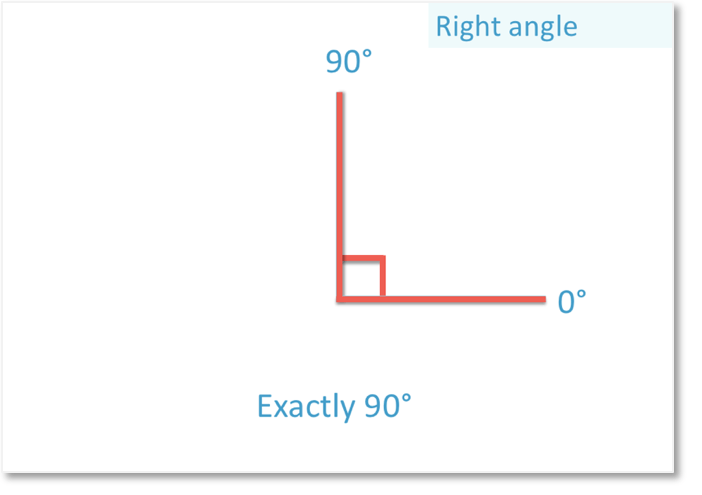Right angles are commonly found around the home such as on the corners of tables, shelves, books and boxes. For this reason, they can be one of the easiest types of angle to teach first.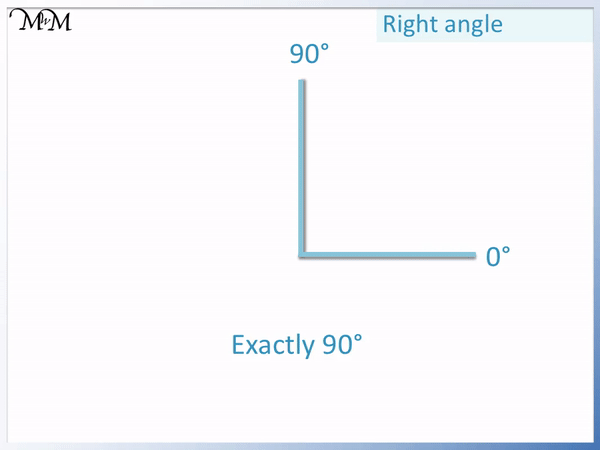The name of right angle orignally comes from the latin words for it,’angulus rectus’. Rectus means upright. When measured from horizontal position, a right angle is upright and hence the name right angle.

## Obtuse Angles

An obtuse angle is larger than 90° but less than 180°. Therefore an obtuse angle is larger than a right angle but is not as large as a straight line.

Here are some examples of obtuse angles.A common real life example of an obtuse angle is the tip of a roof of a house.

## Straight Angles

A straight angle is exactly 180° and is called this because it looks like a straight line. When an object rotates through a straight angle, it has turned through 180° and therefore it has reversed its original direction.

Here is a picture of a straight angle.A straight angle, or straight line must be exactly 180. If it is slightly more or less than this, then there will be a deviation at the corner of the angle and it will not be a straight line.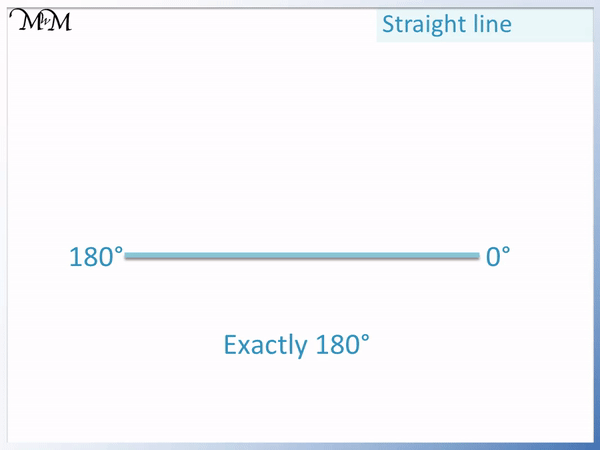Straight lines are found everywhere and children may find it difficult to think of straight line angles as angles rather than lines. To teach straight line angles, it is helpful to mark the centre of the straight line as the corner of the angle. It is also useful to show an angle rotating around between 179° and 181°. In between must be 180°.

## Reflex Angles

Reflex angles are larger than 180° and less than 360°. This means that reflex angles open wider than a straight line but are not large enough to complete a full turn.

Here are some examples of reflex angles.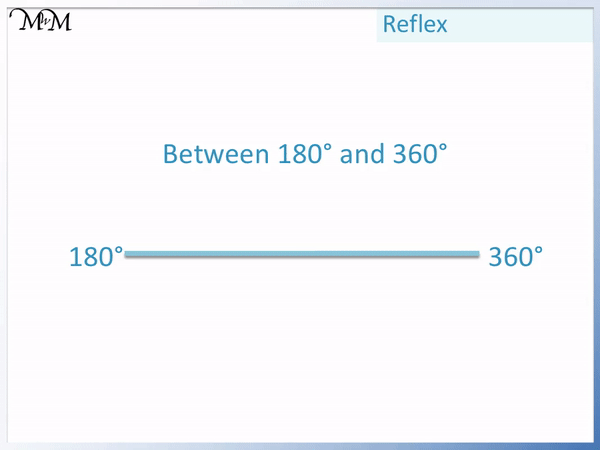Reflex angles are sometimes confused in name with obtuse angles. These are the two most commonly mixed up names when teaching angle classification. It is useful to show examples of both reflex and obtuse angles when teaching them. It can be helpful to compare both angles to a straight line, perhaps using a ruler, which can help us decide if the angle is less than or larger than 180°.

## Full Turns

A full turn is exactly 360°. After completing a full turn, an object is facing the same direction that it was originally.

Here is a diagram showing a full rotation.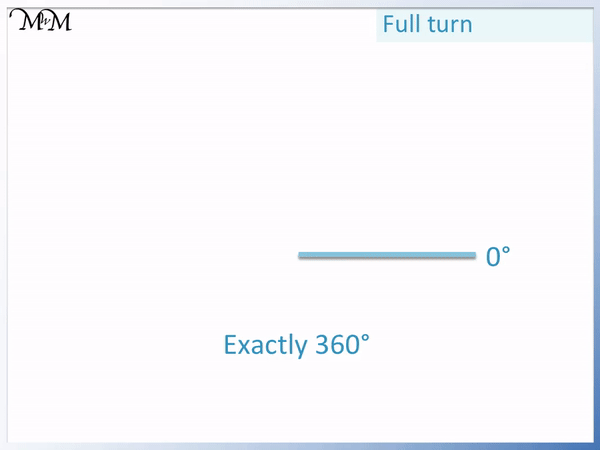Full rotations can sometimes hard to draw or explain since the two arms of the angle are directly on top of each other.Now try our lesson on Why Angles in a Triangle Add to 180 Degrees where we learn about angles in a triangle.